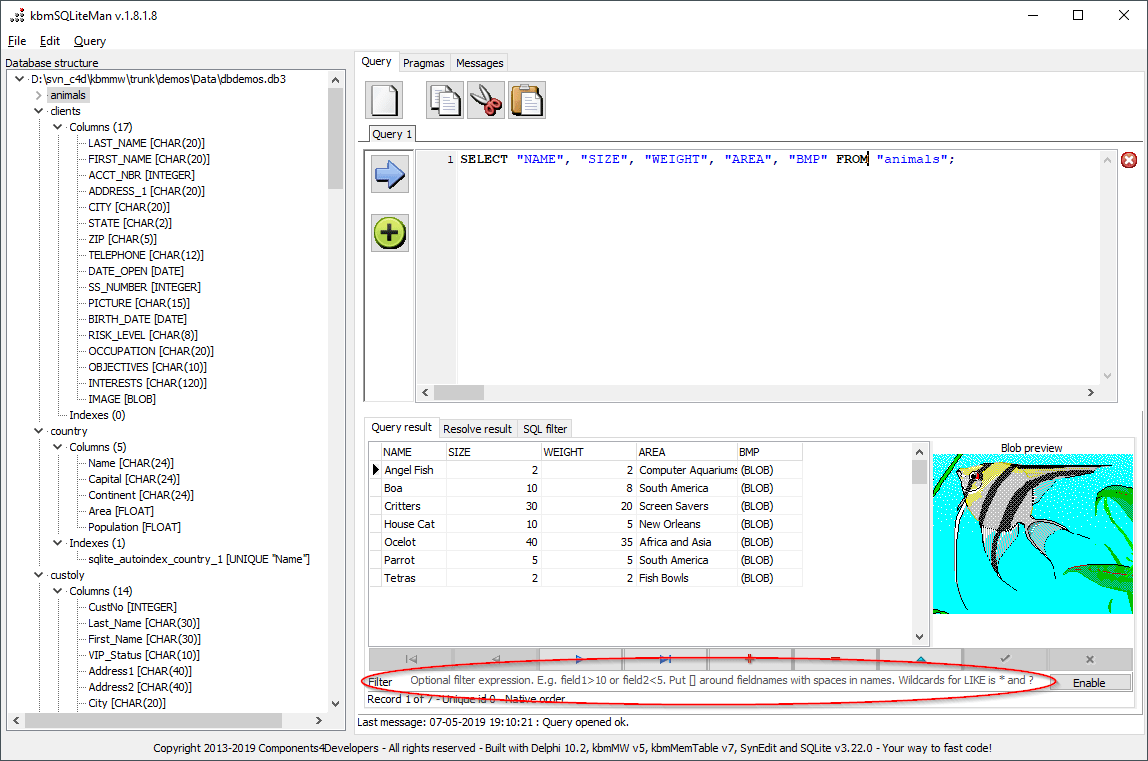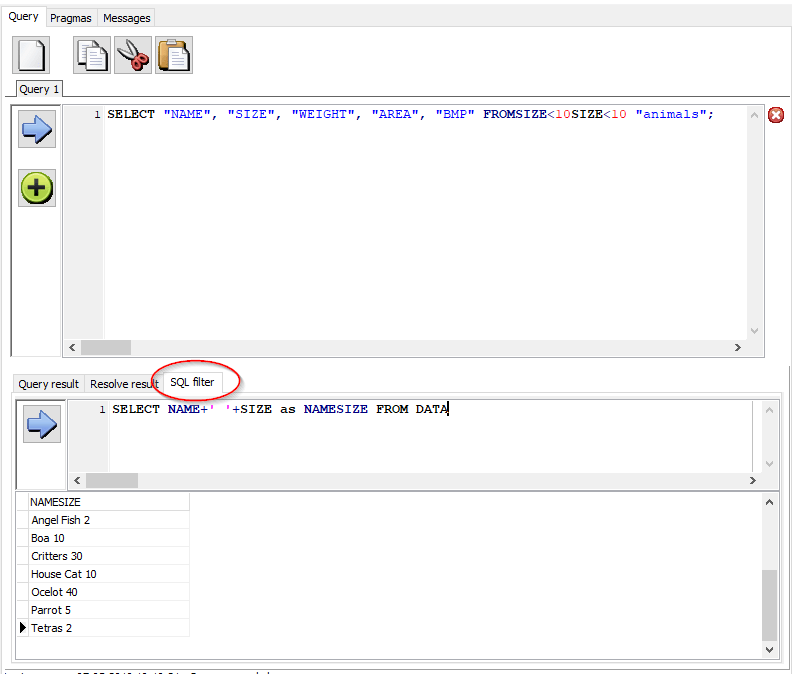# ANN: kbmSQLiteMan v. 1.80 released!Our free cool tool for managing SQLite databases has been released in v. 1.80.

This includes a new local SQL filter capability in addition to being compiled with latest version of kbmMW and kbmMemTable.

### The existing filtering capability

kbmSQLiteMan has for a long time had the ability to locally add an additional filter on result sets returned from the SQLite database.In this filter are, a fairly complex expression can be type, ranging from simple SIZE<10 which filters the result set to only show the 3 records with a SIZE value less than 10. AND, OR, NOT, (), LIKE “P*” etc. are all supported.### The new local SQL “filtering” capability

The above filtering is fine for simply ensuring some records in the result set are not displayed. However if you want to do something more complex with the result set, like complex filtering, grouping, calculations or custom ordering etc, you may want to use the new SQL filtering capability. It internally use kbmMemTable’s SQL ability which is fairly close to regular SQL.Here we combine the result set’s NAME and SIZE column to one column called NAMESIZE. This simple example could of course just as well have been handled in the original SQLite SQL statement, but there may be situations where you want to keep the original data, but do something more with it.

kbmMemTable SQL supports SELECT statements with optional sub select, CASE/WHEN/ELSE, IN, BETWEEN, LIKE, MOD, DIV, ORDER BY, GROUP BY, HAVING, LIMIT, OFFSET, || (concat), +, -, *, / in addition to a good number of functions:

SIN(x), COS(x), TAN(x), LOG(x), LOG2(x), EXP(x), TRUNC(x), FRAC(x), MOD(x), DIV(x), SQRT(x), SQR(x), ROOT(x,y), MIN(x,…), MAX(x,…), AVG(x,…), SUM(x,…), ABS(x), POW(x,y)

UPPER(x), LOWER(x), TRIM(x), TRIMLEFT(x), TRIMRIGHT(x), MID(x,p,n), LEFT(x,n), RIGHT(x,n), LENGTH(x), LEFTPAD(x,c,n), RIGHTPAD(x,c,n), CHR(x), POS(sx,x), REPLACE(x,so,sr [,o1][,o2]), SPLIT(x,sx, OUT v), REGEXP(re,x [,OUT v]),

NOW, DATE(x), TIME(x), YEAR(x), MONTH(x), DAY(x), HOURS(x), MINUTES(x), SECONDS(x), DATESTRING(x), TIMESTRING(x)

CASTTODATETIME(x), CASTTOSTRING(x), CASTTONUMBER(x), CASTTOFLOAT(x), CASTTOINT(x)

IF(x,te,fe [,ne]), NULLIF(x1,x2), ISNULL(x), COALESCE(x,…)

FIELDVALUE(fn), FIELDEXISTS(fn)

```x and y = A value (constant, out defined variable, expression or a field). Values are auto converted between numbers and strings as needed
p = An offset. 1 is first character
n = A number indicating a count
c = A character
sx = A sub string value
so = String to search for
sr = String to replace with
o1 = True/false value. If true, replaces all occurrences
o2 = True/false value. If true, ignores case
v = Name of a variable. The name must start with \$. \$VAR1 is a valid name
re = Regular expression
te = True expression. Returned if x evaluates to true
fe = False expression. Returned if x evaluates to false
ne = NULL expression. Returned if x evaluates to NULL
x1 = A value. If x1=x2, NULL is returned, else x1.
x2 = A value
fn = A fieldname as a string, to return the value from
```

The FROM clause must always refer to the virtual table named DATA which represents the complete original result set.

Examples of valid SQL filter statements

```SELECT fld5,MAX(fld1),MAX(fld2) FROM DATA GROUP BY fld5
SELECT DISTINCT * FROM DATA
SELECT FieldValue('fld1') as myfld1,Coalesce(FieldValue('NoField'),"ISNULL") as NoField  FROM DATA
SELECT Coalesce(10) FROM DATA
SELECT Coalesce(Null) FROM DATA
SELECT Coalesce(fld7,99999) FROM DATA
SELECT Coalesce(fld7,Null,99999) FROM DATA
SELECT fld1, (SELECT Max(fld1) FROM DATA) FROM DATA
SELECT fld1 FROM DATA WHERE fld3 IN (SELECT b.fld2 FROM DATA b WHERE b.fld2<4)
SELECT 1, MAX(fld1) FROM DATA GROUP BY 1
SELECT 1 AS 'ID', MAX(fld1) FROM DATA GROUP BY ID
SELECT NULL as fld1, fld2, "ABC" as fld3, 10+20 as fld4 FROM DATA
SELECT RegExp("1\d",fld2), fld2 FROM DATA
SELECT RegExp("^(\d)(\d*)",fld2,OUT \$PAR),\$PAR,fld2 FROM DATA
SELECT fld1 AS TIME, fld2 FROM DATA WHERE fld1 LIKE "STR1%"
SELECT fld1, fld2 FROM DATA WHERE fld1 LIKE "STR1%"
SELECT fld1, fld2 FROM DATA WHERE NOT fld1 LIKE "STR1%"
SELECT fld1, fld2 FROM DATA WHERE fld1 NOT LIKE "STR1%"
SELECT fld1, fld2 FROM DATA WHERE fld2 LIKE "8%"
SELECT fld1, fld2, CASE WHEN fld2<100 THEN 'LOW' WHEN fld2>=100 AND fld2<200 THEN 'MEDIUM' ELSE 'HIGH' END FROM DATA
SELECT fld1, fld2, CASE fld2 WHEN 10 THEN 99999 WHEN 20 THEN 22222 ELSE -1 END FROM DATA
SELECT RecNo,RowID,* FROM DATA
SELECT fld1 FROM DATA WHERE fld2 in (10,20,30)
SELECT 1-2-3 FROM DATA LIMIT 1
SELECT fld2+1 as fld2a FROM DATA ORDER BY fld2a DESC
SELECT fld2+1 as fld2 FROM DATA
SELECT fld1,fld2,fld3,fld6,fld3 AS SomeField1,fld2 AS SomeField2,fld5 FROM DATA WHERE fld5 IN (5) ORDER BY fld6,SomeField2
SELECT fld2 as Field2, fld3, sum(fld5) as fld5, Sum(fld2) as SomeField1, Sum(fld3) as SomeField2 FROM DATA GROUP BY Field2, fld3
SELECT fld2 as Field2, fld3, sum(fld5) as SomeField1, Sum(fld2) as SomeField2, Sum(fld3) as SomeField3 FROM DATA GROUP BY Field2, fld3
SELECT fld5,sum(fld5) as sumoffld5,count(fld5) as countoffld5 FROM DATA GROUP BY fld5 HAVING count(fld5)>2
SELECT fld2 as somefield, fld3 FROM DATA
SELECT fld5 as somefield,sum(fld5),count(fld5) FROM DATA GROUP BY somefield HAVING count(fld5)>2
SELECT count(*)+5 FROM DATA
SELECT * FROM DATA LIMIT 10 OFFSET 50
SELECT * FROM DATA LIMIT 10
SELECT * FROM DATA OFFSET 50
SELECT fld2, IF(fld2>10,True,False) AS IsSomething FROM DATA
SELECT SUM(fld5),SUM(fld6),SUM(fld5)+Sum(fld6) AS TotalField FROM DATA
SELECT count(distinct Left(fld1,4)) from DATA
SELECT length(fld1) from DATA
SELECT fld5,sum(if(fld5>5,1,0)),count(fld5) from DATA group by fld5
SELECT fld1,Min(20,30,10,40),Max(20,30,10,40),Avg(20,30,10,40),Sum(20,30,10,40)  FROM DATA
SELECT fld2 FROM DATA where fld2 xor (fld2 mod 10)
SELECT if(1 xor 1,0,1),fld2 FROM DATA
SELECT if(0 xor 1,0,1),fld2 FROM DATA
SELECT if(10 IN (10,20,30),1,0),fld2 FROM DATA
SELECT if(11 IN (10,20,30),1,0),fld2 FROM DATA
SELECT MAX((SELECT max(b.fld1) FROM DATA b WHERE a.fld2=b.fld2)) FROM DATA a
```

Happy filtering with the new kbmSQLiteMan release!

As usual… if you like our products and what you read, please share the blog posts with whom you know.
Without you C4D will not produce great products!

16,398 total views,  1 views today

This site uses Akismet to reduce spam. Learn how your comment data is processed.